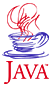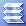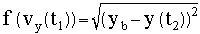Home : Map : Chapter 4 : Java : Tech : Physics : Demo 3: Shooting at a TargetIntroduction Inheritance   Demo 1 Overriding   Demo 2a   Demo 2b this,super MoreConstructors   Demo 3 Abstraction Interface    Demo 4 Casting References MoreAboutArrays Object class Class Summary Exercises
 Supplements Override/Overload Annotation-J2SE5.0 Java Security Class Loading Class Verifier SecurityManager
 About JavaTech      Codes List      Exercises      Feedback      References      Resources      Tips      Topic Index      Course Guide      What's New

This demo applies the shooting method to the actual task of literally shooting a projectile at a target. The projectile begins from a given point and a given horizontal velocity. The shooting method must determine the inital vertical velocity component (vy_init) that will send the projectile to hit the target vertical point after traveling a 10 seconds.

A function (or score) is created using distance of the target to the final vertical value of the projectile. The discrete Newton (or secant) method is used to determine the root of this function.

For each vy_init point a 4th order Runge-Kutta integration of the trajectory is carried out. The Newton method needs to calculate trajectory twice initially and then once for each iteration.

For the projectile there are 4 (=N) variables dependent on t :

x(t)  - horizontal coordinate
y(t)  - vertical coordinate
vx(t) - horizontal velocity
vy(t) - vertical velocity

Here we set three (=n) variables to fixed initial values:

x(t1),y(t1) - initial location
vx(t1) - initial horizontal velocity

We guess at an initial value for the free variable

vy(t1)

One (=m) of the four variables has fixed final values:

yb - target point

The final velocities are not specified.

The program varies vy(t1) such that the final y values match the target point. The discrete Newton (secant) method is used to find the root ofThe following code shows the Shoot_Applet6.java code that carries out the shooting algorithm. The helper class Shooter below does the trajectory finding with the 4th order Runge-Kutta :

ShootApplet.java
(Output goes to browser's Java console.)
/**  Program using the shooting method to find the initial
*  vertical velocity component of a projectile such that it
*  hits a target point.
**/
public class ShootApplet extends java.applet.Applet
{
// Parameters for the algorithms
double dvy = 1.0;
double tol = 0.1;
double dt  = 0.001;

int nSteps = 10000; // => 10 seconds of flight
int maxSteps = 2000;

double x_init   = 0.0;
double y_init   = 0.0;
double vx_init  = 400.0;
double vy_init  = 1.0;// first guess

// Set target point
double posTarget = 100.0;

// Create an instance of the shooting algorithm class.
Shooter shooter = new Shooter ();

// These arrays hold the coordinates and velocities
// of the projectile at start and end.
double [] pos_final  = new double ;
double [] pos_init   = new double ;
double [] v_init     = new double ;
double [] v_final    = new double ;

/** Set up and then use the shooting method to determine
* the initial velocities that led to a projectile hitting
* the given target.
**/
public void init () {
// Set initial conditions.
pos_init   = x_init;
pos_init   = y_init;
v_init     = vx_init;
v_init     = vy_init;

// At step n, these variables hold the
// nth, n-1, and n-2 values for the velocity
// and scores.
double vyn, vyn_1, vyn_2;
double fn, fn_1, fn_2;

// Discrete Newton method needs 2 initial points.
vyn_2 = v_init; // First guess at vy_init
fn_2 = f ();

v_init += dvy;  // Second guess at vy_init
vyn_1 = v_init;
fn_1 = f ();

int numSteps = 0;
do {
double divisor = (fn_1 - fn_2);
if (divisor == 0.0) {
System.out.println ("Derivative invalid");
return;
}
// Calculate new estimate of the vy_init
// using the discrete Newton  (i.e. secant) method
double del = (vyn_1 - vyn_2) * (fn_1)/divisor;
System.out.println (" del = " + del);
vyn = vyn_1 - del;
v_init = vyn; // new vy inital estimate

// Calculate trajectory with this new initial vy
// and return a "score" for how close the shot came
// to the target.
fn = f ();

// Save the past two estimates of the velocity
vyn_2 = vyn_1;
vyn_1 = vyn;

// Save past two score values.
fn_2 = fn_1;
fn_1 = fn;

numSteps++;
} while  (fn > tol && numSteps < maxSteps);

System.out.println ();
System.out.println ("After steps = "+numSteps);
System.out.println ("  & Newton steps = "+numSteps);
System.out.println ("Root vy_init = "+v_init+
" results in a distance = "+fn+
" from target");
}

/** Calculate the distance between the end point of the
* trajectory of the projectile (as calculated from the
* Runge-Kutta method) and the desired target point.
**/
double f () {

// Shooter uses the Runge-Kutta method to step
// the projectile through a trajectory using
// the initial values passed here.
shooter.shoot (pos_init, v_init,
pos_final,v_final,
dt, nSteps);

double val =
(posTarget-pos_final)*
(posTarget-pos_final);
return Math.sqrt (val);
} // f

/** Paint message in Applet window. **/
public void paint (java.awt.Graphics g) {
g.drawString ("ShootApplet",20,20);
}

/** Run optionally as an application. **/
public static void main (String [] args) {
ShootApplet obj = new ShootApplet ();
obj.init ();
} // main

} // ShootApplet

The Shooter class implements the Derivable class discussed earlier. The stopping point for the integration comes when the horizontal position of the target is passed. An interpolation back to the vertical plane at xb is carried out to get a better estimate for the crossing point.

 Shooter.java /** Shoot carries out a Runge-Kutta integration of a   *  projectile trajectory.  **/ public class Shooter implements Derivable {   // Constants   static double g  = 9.80;// meter per sec**2   // Instance variables   double [] pos = new double;   double [] vel = new double;   // Method to carry out trajectory calculation   void shoot (double [] pos_init,               double [] v_init,               double [] pos_final,               double [] v_final,               double dt, int nSteps) {     double t = 0.0;     int n;     pos = pos_init; // x     pos = pos_init; // y     vel = v_init;   // vx     vel = v_init;   // vy     for (n=0; n < nSteps; n++) {       RungeKutta4th.step (t,dt,pos,vel,this);     }     pos_final = pos;     pos_final = pos;     v_final = vel;     v_final = vel;     System.out.println (" In Shooter after steps =" + n);     System.out.println (" x,y = " + pos + ", " + pos);   } // shoot   /** Implement the interface method for the derivative     * of the variables. **/   public double deriv (int i, double var, double vel, double t) {     if (i == 0) { // x variable         return 0.0;     } else { // y variable         return -g;     }   } // deriv } // Shooter

For this case, only one interation of the Newton algorithm is needed to find the root as this output shows:

 In Shooter after steps =10000 x,y = 4000.0000000006353, -479.99999999995055 In Shooter after steps =10000 x,y = 4000.0000000006353, -469.9999999999515 del = -57.00000000000099 In Shooter after steps =10000 x,y = 4000.0000000006353, 100.00000000006408 After steps = 1 & Newton steps = 1 Root vy_init = 59.00000000000099 results in a distance = 6.407674391084583E-11 from target

Exercise: Try some other values for the target, the starting values, the increment sizes, etc to see that the program still finds the target and find how quickly the method finds the solution.

Most recent update: Oct. 21, 2005

 Tech MoreComplexClass ImprovedHistogram JavaRandomNums Vectors & Matrices Exercises
 Physics Runge-Kutta 2nd   Demo 1 Runge-Kutta 4th   Demo 2 BoundaryVal.Prob Shooting Method   Demo 3 ExercisesPart I Part II Part III Java Core 1  2  3  4  5  6  7  8  9  10  11  12 13 14 15 16 17 18 19 20 21 22 23 24 Supplements 1  2  3  4  5  6  7  8  9  10  11  12 Tech 1  2  3  4  5  6  7  8  9  10  11  12 Physics 1  2  3  4  5  6  7  8  9  10  11  12

Java is a trademark of Sun Microsystems, Inc.